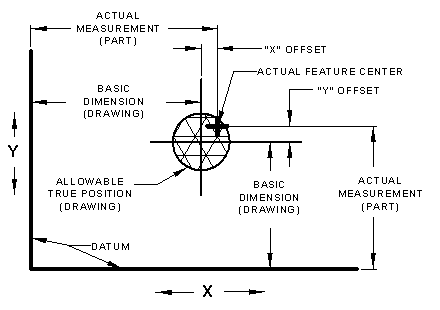#### True Position GD&T Tolerance Calculator

Calculators | Technology Store

(This calculator requires a java - enabled browser)

This calculator calculates position tolerances utilizing principles and concepts withinASME Y14.5-2009 and ASME Y14.5M - 1994, Geometric Dimensioning and Tolerancing (GD&T).

For the equations behind this calculator, see:
"Geometric Boundaries"
Interpretation and Application of
Geometric Dimensioning and Tolerancing

Negative Tolerance numbers indicate out of tolerance condition.

This GD&T true position calculator will convert coordinate measurements to position tolerances. Two (2) inputs are required, See the application illustration at bottom of page.

The following are definitions for the elements:

Offset (X) - This is the difference between the basic dimension (Drawing) and the actual measurement of the produced part.

Offset (Y) - This is the difference between the basic dimension (Drawing) and the actual measurement of the produced part.

Calculated (ACTUAL) True Position - The calculated positional tolerance diameter zone.

Allowable True Position - This is the true position tolerance defined/required (drawing) .

(+) Excess Tolerance - Additional available positional tolerance.

(-) Excess Tolerance - Allowed positional tolerance exceed by given measurements .Related Resources: